# Electronics and Communication Engineering - Analog Electronics

21.

The circuit shown is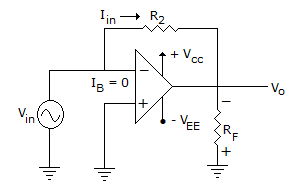A. voltage series feedback amplifier B. transconductance amplifier C. transresistance amplifier with open circuit mutual resistance RF D. transresistance amplifier with short circuit mutual resistance RF

Explanation:

Circuit can be redrawn as.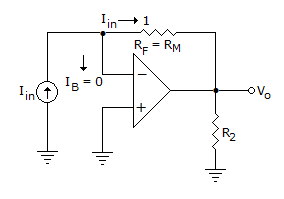This is an inverting amplifier with inverting terminal virtual ground.

Hence, Vo = - iin RF

This RF is termed as mutual resistance here, Rm.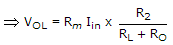Where R0 is output Resistance of Op-Amp.

if RL >> R0 output terminals become open circuited.

and VoL = Rm.Iin

where Rm work as mutual resistance.

22.

Consider 49 cascaded amplifiers having individual rise time as 2 n sec. 3 n sec. ... 50 n sec. The input waveform rise time is 1 n sec. Then the output signal rise time is given time by (Assume output signal rise time is measured within 10 percent range of the final output signal.)

 A. 39 - 28 μsec B. 2.28 μsec C. 0.228 μsec D. 39.28 μsec

Explanation:

Output rise time (tr)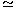1.1ti02 + t12 + ... + t492,

where t, t2 ... t49 are the individual rise time.tr1.1 (1 ns)2 + (2 ns)2 + (50 ns)21.1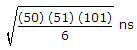0.228 msec.

23.

The zener diode in the rectangular circuit shown in the figure has a zener voltage of 5.8 volts and a zener knee current of 0.5 mA. The maximum load current drawn from this circuit ensuring proper functioning over the input voltage range between 20 and 30 volts, is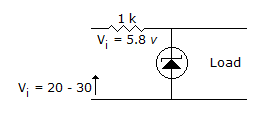A. 23.7 mA B. 14.2 mA C. 13.7 mA D. 24.2 mA

Explanation:

It is zener diode, hence 5.8 volt remain constant.By applying KVL30 = 1000 Imax + 5.8Imax = 24.2 mA.

24.

In figure, ID = 4 mA. Then VDS =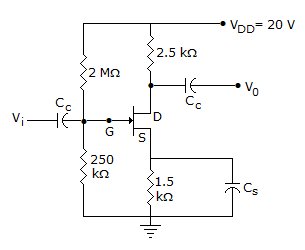A. 20 V B. 16 V C. 4 V D. 0.4 V

Explanation:

VDS = VD - VS = 10-6 = 4 V.

25.

In figure, V0 =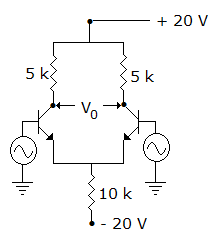A. 20 V B. 10 V C. 5 V D. 0